### Ninja Star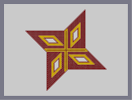Hover over the thumbnail for a full-size version.

Author DragonCypher author:dragoncypher n-art rated 2005-12-03 4 by 13 people. \$Ninja Star#DragonCypher#none#00000000000000000000000000000000000000000000000000000000000000000000000000000000000000000000000000000000000000000000000000000000000000000000000000000000000000000000000000000000000000000000000000000000000000000000000000000000000000000000000000000000000000000000000000000000000000000000000000000000000000000000000000000000000000000000000000000000000000000000000000000000000000000000000000000000000000000000000000000000000000000000000000000000000000000000000000000000000000000000000000000000000000000000000000000000000000000000000000000000000000000000000000000000000000000000000000000000000000000000000000000000000000000000000000000000000000000000000000000000000000000000000000000000000000000000000000000000000000000|12^282,312!12^282,318!12^282,324!12^282,330!12^282,336!12^282,342!12^282,348!12^282,354!12^282,360!12^282,366!12^282,372!12^282,378!12^282,384!12^282,390!12^282,396!12^282,402!12^282,408!12^282,414!12^282,420!12^282,426!12^282,432!12^282,438!12^282,444!12^282,450!12^282,456!12^282,462!12^282,468!12^282,474!12^282,480!12^282,486!12^282,492!12^282,498!12^282,504!12^282,510!12^282,516!12^282,522!12^282,528!12^282,534!12^282,540!12^396,300!12^408,414!12^414,414!12^420,414!12^426,414!12^432,414!12^438,414!12^444,414!12^450,414!12^456,414!12^462,414!12^468,414!12^474,414!12^480,414!12^486,414!12^492,414!12^498,414!12^504,414!12^510,414!12^516,414!12^522,414!12^528,414!12^534,414!12^540,414!12^546,414!12^552,414!12^558,414!12^564,414!12^570,414!12^576,414!12^582,414!12^588,414!12^594,414!12^600,414!12^606,414!12^612,414!12^618,414!12^624,414!12^630,414!12^636,414!12^510,288!12^510,276!12^510,282!12^510,270!12^510,264!12^510,252!12^510,258!12^510,246!12^510,240!12^510,234!12^510,228!12^510,222!12^510,216!12^510,210!12^510,204!12^510,198!12^510,192!12^510,186!12^510,180!12^510,174!12^510,168!12^510,162!12^510,156!12^510,150!12^510,144!12^510,138!12^510,132!12^510,126!12^510,120!12^510,114!12^510,108!12^510,102!12^510,96!12^510,90!12^510,84!12^510,78!12^510,72!12^510,66!12^510,60!12^384,186!12^378,186!12^372,186!12^366,186!12^360,186!12^354,186!12^348,186!12^342,186!12^336,186!12^330,186!12^324,186!12^318,186!12^312,186!12^306,186!12^300,186!12^294,186!12^288,186!12^282,186!12^276,186!12^270,186!12^264,186!12^258,186!12^252,186!12^246,186!12^240,186!12^234,186!12^228,186!12^222,186!12^216,186!12^204,186!12^210,186!12^198,186!12^192,186!12^186,186!12^180,186!12^174,186!12^168,186!12^162,186!12^156,186!12^504,66!12^498,72!12^492,78!12^486,84!12^480,90!12^474,96!12^468,102!12^462,108!12^456,114!12^450,120!12^444,126!12^438,132!12^432,138!12^426,144!12^420,150!12^414,156!12^408,162!12^402,168!12^396,174!12^390,180!12^162,192!12^168,198!12^174,204!12^180,210!12^186,216!12^192,222!12^198,228!12^204,234!12^210,240!12^216,246!12^222,252!12^228,258!12^234,264!12^240,270!12^246,276!12^252,282!12^258,288!12^264,294!12^270,300!12^276,306!12^288,534!12^294,528!12^300,522!12^306,516!12^312,510!12^318,504!12^324,498!12^330,492!12^336,486!12^342,480!12^348,474!12^354,468!12^360,462!12^366,456!12^372,450!12^378,444!12^384,438!12^390,432!12^396,426!12^402,420!12^630,408!12^624,402!12^618,396!12^612,390!12^606,384!12^600,378!12^594,372!12^588,366!12^582,360!12^576,354!12^570,348!12^564,342!12^558,336!12^552,330!12^546,324!12^540,318!12^534,312!12^528,306!12^522,300!12^516,294!12^396,294!12^396,288!12^396,282!12^396,276!12^396,270!12^396,264!12^396,258!12^396,252!12^396,246!12^396,240!12^396,234!12^396,228!12^396,222!12^396,216!12^396,210!12^396,204!12^396,198!12^396,192!12^396,186!12^396,180!12^402,300!12^408,300!12^414,300!12^426,300!12^420,300!12^432,300!12^438,300!12^444,300!12^450,300!12^456,300!12^462,300!12^468,300!12^474,300!12^480,300!12^486,300!12^492,300!12^498,300!12^504,300!12^510,300!12^516,300!12^396,306!12^396,312!12^396,318!12^396,324!12^396,330!12^396,336!12^396,342!12^396,348!12^396,354!12^396,360!12^396,366!12^396,372!12^396,378!12^396,384!12^396,390!12^396,396!12^396,402!12^396,408!12^396,414!12^396,420!12^390,300!12^384,300!12^378,300!12^372,300!12^366,300!12^360,300!12^354,300!12^348,300!12^342,300!12^336,300!12^330,300!12^324,300!12^318,300!12^312,300!12^306,300!12^300,300!12^294,300!12^288,300!12^282,300!12^276,300!0^432,252!0^432,246!0^432,240!0^432,234!0^432,228!0^432,222!0^432,216!0^432,210!0^432,204!0^432,198!0^438,192!0^444,186!0^450,180!0^456,174!0^462,168!0^468,162!0^474,156!0^432,264!0^432,258!0^474,162!0^474,168!0^474,174!0^474,180!0^474,186!0^474,192!0^474,198!0^438,258!0^444,252!0^450,246!0^456,240!0^462,234!0^468,228!0^474,222!0^474,216!0^474,210!0^474,204!0^432,270!0^438,264!0^444,258!0^450,252!0^456,246!0^462,240!0^468,234!0^474,228!0^480,222!0^480,216!0^480,210!0^480,204!0^480,198!0^480,192!0^480,186!0^480,180!0^480,174!0^480,168!0^480,162!0^480,156!0^480,150!0^480,144!0^474,150!0^468,156!0^462,162!0^456,168!0^450,174!0^444,180!0^438,186!0^432,192!0^426,198!0^426,204!0^426,210!0^426,216!0^426,222!0^426,228!0^426,234!0^426,240!0^426,246!0^426,252!0^426,258!0^426,264!0^426,270!0^426,276!0^432,276!0^438,270!0^426,282!0^444,264!0^450,258!0^456,252!0^462,246!0^468,240!0^474,234!0^480,228!0^426,192!0^432,186!0^438,180!0^444,174!0^450,168!0^456,162!0^462,156!0^468,150!0^474,144!0^480,138!0^510,294!0^504,294!0^498,294!0^492,294!0^486,294!0^480,294!0^474,294!0^468,294!0^462,294!0^456,294!0^450,294!0^444,294!0^438,294!0^432,294!0^426,294!0^420,294!0^414,294!0^408,294!0^402,294!0^402,288!0^402,282!0^402,276!0^402,270!0^402,264!0^402,258!0^402,252!0^402,246!0^402,240!0^402,234!0^402,228!0^402,222!0^402,216!0^402,210!0^402,204!0^402,198!0^402,192!0^402,186!0^402,180!0^402,174!0^390,186!0^390,192!0^390,198!0^390,204!0^390,210!0^390,216!0^390,222!0^390,228!0^390,234!0^390,240!0^390,246!0^390,252!0^390,258!0^390,264!0^390,270!0^390,276!0^390,282!0^390,288!0^390,294!0^384,294!0^378,294!0^372,294!0^366,294!0^360,294!0^354,294!0^348,294!0^342,294!0^336,294!0^330,294!0^324,294!0^318,294!0^312,294!0^306,294!0^300,294!0^294,294!0^288,294!0^282,294!0^276,294!0^270,294!0^282,306!0^288,306!0^294,306!0^300,306!0^306,306!0^312,306!0^318,306!0^324,306!0^330,306!0^336,306!0^342,306!0^348,306!0^354,306!0^360,306!0^366,306!0^372,306!0^378,306!0^384,306!0^390,306!0^390,312!0^390,318!0^390,324!0^390,330!0^390,336!0^390,342!0^390,348!0^390,354!0^390,360!0^390,366!0^390,372!0^390,378!0^390,384!0^390,390!0^390,396!0^390,402!0^390,408!0^390,414!0^390,420!0^390,426!0^402,414!0^402,408!0^402,402!0^402,396!0^402,390!0^402,384!0^402,378!0^402,372!0^402,366!0^402,360!0^402,354!0^402,348!0^402,342!0^402,336!0^402,330!0^402,324!0^402,318!0^402,312!0^402,306!0^408,306!0^414,306!0^420,306!0^426,306!0^432,306!0^438,306!0^444,306!0^450,306!0^456,306!0^462,306!0^468,306!0^474,306!0^480,306!0^486,306!0^492,306!0^498,306!0^504,306!0^510,306!0^516,306!0^522,306!12^438,252!12^444,246!12^450,240!12^456,234!12^462,228!12^468,222!12^468,216!12^468,210!12^468,204!12^468,198!12^468,192!12^468,186!12^468,180!12^468,174!12^468,168!12^462,174!12^456,180!12^450,186!12^444,192!12^438,198!12^438,204!12^438,210!12^438,222!12^438,216!12^438,228!12^438,234!12^438,240!12^438,246!12^504,288!12^504,282!12^504,276!12^504,270!12^504,264!12^504,258!12^504,252!12^504,246!12^504,240!12^504,234!12^504,228!12^504,222!12^504,216!12^504,210!12^504,204!12^504,198!12^504,192!12^504,186!12^504,180!12^504,174!12^504,168!12^504,162!12^504,156!12^504,150!12^504,144!12^504,138!12^504,126!12^504,132!12^504,120!12^504,114!12^504,108!12^504,102!12^504,96!12^504,90!12^504,84!12^504,78!12^504,72!12^498,78!12^498,84!12^498,90!12^498,96!12^498,102!12^498,108!12^498,114!12^498,120!12^498,126!12^498,132!12^498,138!12^498,144!12^498,150!12^498,156!12^498,162!12^498,168!12^498,174!12^498,180!12^498,186!12^498,192!12^498,198!12^498,204!12^498,210!12^498,216!12^498,222!12^498,228!12^498,234!12^498,240!12^498,246!12^498,252!12^498,258!12^498,264!12^498,270!12^498,276!12^498,282!12^498,288!12^492,288!12^492,282!12^492,276!12^492,270!12^492,264!12^492,258!12^492,252!12^492,246!12^492,240!12^492,234!12^492,228!12^492,222!12^492,216!12^492,210!12^492,204!12^492,198!12^492,192!12^492,186!12^492,180!12^492,174!12^492,168!12^492,162!12^492,156!12^492,150!12^492,144!12^492,138!12^492,132!12^492,126!12^492,120!12^492,114!12^492,108!12^492,102!12^492,96!12^492,90!12^492,84!12^486,90!12^486,96!12^486,102!12^486,108!12^486,114!12^486,120!12^486,126!12^486,132!12^486,138!12^486,144!12^486,150!12^486,156!12^486,162!12^486,168!12^486,174!12^486,180!12^486,186!12^486,192!12^486,198!12^486,204!12^486,210!12^486,216!12^486,222!12^486,228!12^486,234!12^486,240!12^486,246!12^486,252!12^486,258!12^486,264!12^486,270!12^486,276!12^486,282!12^486,288!12^480,288!12^480,282!12^480,276!12^480,270!12^480,264!12^480,258!12^480,252!12^480,246!12^480,240!12^480,234!12^480,132!12^480,126!12^480,120!12^480,114!12^480,108!12^480,102!12^480,96!12^474,102!12^474,108!12^474,114!12^474,120!12^474,126!12^474,132!12^474,138!12^474,240!12^474,246!12^474,252!12^474,258!12^474,264!12^474,270!12^474,276!12^474,282!12^474,288!12^468,288!12^468,282!12^468,276!12^468,270!12^468,264!12^468,258!12^468,252!12^468,246!12^468,144!12^468,138!12^468,132!12^468,126!12^468,120!12^468,114!12^468,108!12^462,114!12^462,120!12^462,126!12^462,132!12^462,138!12^462,144!12^462,150!12^462,252!12^462,258!12^462,264!12^462,270!12^462,276!12^462,282!12^462,288!12^456,288!12^456,282!12^456,276!12^456,270!12^456,264!12^456,258!12^456,156!12^456,150!12^456,144!12^456,138!12^456,132!12^456,126!12^456,120!12^450,126!12^450,132!12^450,138!12^450,144!12^450,150!12^450,156!12^450,162!12^450,264!12^450,270!12^450,276!12^450,282!12^450,288!12^444,288!12^444,282!12^444,276!12^444,270!12^444,168!12^444,162!12^444,156!12^444,150!12^444,144!12^444,132!12^444,138!12^438,138!12^438,144!12^438,150!12^438,156!12^438,162!12^438,168!12^438,174!12^438,276!12^438,282!12^438,288!12^432,288!12^432,282!12^432,180!12^432,174!12^432,168!12^432,162!12^432,156!12^432,150!12^432,144!12^426,150!12^426,156!12^426,162!12^426,168!12^426,174!12^426,180!12^426,186!12^426,288!12^420,288!12^420,282!12^420,276!12^420,270!12^420,264!12^420,258!12^420,252!12^420,246!12^420,240!12^420,234!12^420,228!12^420,228!12^420,222!12^420,216!12^420,210!12^420,204!12^420,198!12^420,192!12^420,186!12^420,180!12^420,174!12^420,168!12^420,162!12^420,156!12^414,162!12^414,168!12^414,174!12^414,180!12^414,186!12^414,192!12^414,198!12^414,204!12^414,210!12^414,216!12^414,222!12^414,228!12^414,234!12^414,240!12^414,246!12^414,252!12^414,258!12^414,264!12^414,270!12^414,276!12^414,282!12^414,288!12^408,288!12^408,282!12^408,276!12^408,270!12^408,264!12^408,258!12^408,252!12^408,246!12^408,240!12^408,234!12^408,228!12^408,222!12^408,216!12^408,210!12^408,204!12^408,198!12^408,192!12^408,186!12^408,180!12^408,174!12^408,168!0^378,270!0^372,270!0^366,270!0^360,270!0^354,270!0^348,270!0^342,270!0^336,270!0^330,270!0^324,270!0^318,270!0^312,270!0^306,270!0^300,270!0^294,270!0^288,270!0^282,264!0^276,258!0^270,252!0^264,246!0^258,240!0^252,234!0^246,228!0^240,222!0^234,216!0^372,264!0^366,258!0^360,252!0^354,246!0^348,240!0^342,234!0^336,228!0^330,222!0^324,216!0^312,216!0^318,216!0^306,216!0^300,216!0^294,216!0^288,216!0^282,216!0^276,216!0^270,216!0^264,216!0^258,216!0^252,216!0^246,216!0^240,216!0^246,222!0^252,228!0^258,234!0^264,240!0^270,246!0^276,252!0^282,258!0^288,264!0^294,264!0^300,264!0^306,264!0^312,264!0^318,264!0^324,264!0^330,264!0^336,264!0^342,264!0^348,264!0^354,264!0^360,264!0^366,264!0^360,258!0^354,252!0^348,246!0^342,240!0^336,234!0^330,228!0^324,222!0^318,222!0^312,222!0^306,222!0^300,222!0^294,222!0^288,222!0^282,222!0^276,222!0^270,222!0^264,222!0^258,222!0^252,222!0^258,228!0^264,234!0^270,240!0^276,246!0^282,252!0^288,258!0^354,258!0^348,252!0^342,246!0^336,240!0^330,234!0^324,228!12^348,258!12^342,252!12^336,246!12^330,240!12^324,234!12^318,228!12^312,228!12^306,228!12^300,228!12^294,228!12^288,228!12^282,228!12^276,228!12^270,228!12^264,228!12^270,234!12^276,240!12^282,246!12^288,252!12^294,258!12^300,258!12^306,258!12^312,258!12^318,258!12^324,258!12^330,258!12^336,258!12^342,258!12^384,192!12^378,192!12^372,192!12^366,192!12^360,192!12^354,192!12^348,192!12^342,192!12^336,192!12^330,192!12^324,192!12^318,192!12^312,192!12^306,192!12^300,192!12^288,192!12^294,192!12^282,192!12^276,192!12^270,192!12^264,192!12^258,192!12^252,192!12^246,192!12^240,192!12^228,192!12^234,192!12^222,192!12^216,192!12^210,192!12^198,192!12^204,192!12^192,192!12^186,192!12^180,192!12^174,192!12^168,192!12^174,198!12^180,198!12^186,198!12^192,198!12^198,198!12^204,198!12^210,198!12^216,198!12^222,198!12^228,198!12^234,198!12^240,198!12^246,198!12^252,198!12^258,198!12^264,198!12^270,198!12^276,198!12^282,198!12^288,198!12^294,198!12^300,198!12^306,198!12^312,198!12^318,198!12^324,198!12^330,198!12^336,198!12^342,198!12^348,198!12^360,198!12^354,198!12^366,198!12^372,198!12^378,198!12^384,198!12^384,204!12^378,204!12^372,204!12^360,204!12^366,204!12^354,204!12^348,204!12^342,204!12^336,204!12^330,204!12^324,204!12^318,204!12^312,204!12^306,204!12^300,204!12^294,204!12^288,204!12^282,204!12^276,204!12^270,204!12^264,204!12^258,204!12^252,204!12^246,204!12^240,204!12^234,204!12^228,204!12^222,204!12^210,204!12^216,204!12^204,204!12^198,204!12^192,204!12^186,204!12^180,204!12^186,210!12^192,210!12^198,210!12^204,210!12^210,210!12^216,210!12^222,210!12^228,210!12^234,210!12^240,210!12^246,210!12^252,210!12^258,210!12^264,210!12^270,210!12^276,210!12^282,210!12^288,210!12^294,210!12^300,210!12^306,210!12^312,210!12^318,210!12^324,210!12^330,210!12^336,210!12^342,210!12^348,210!12^354,210!12^360,210!12^366,210!12^378,210!12^372,210!12^384,210!12^384,216!12^378,216!12^372,216!12^366,216!12^360,216!12^354,216!12^348,216!12^342,216!12^336,216!12^330,216!12^228,216!12^222,216!12^216,216!12^210,216!12^204,216!12^198,216!12^192,216!12^384,222!12^378,222!12^372,222!12^366,222!12^360,222!12^354,222!12^348,222!12^342,222!12^336,222!12^234,222!12^228,222!12^222,222!12^216,222!12^204,222!12^210,222!12^198,222!12^204,228!12^210,228!12^216,228!12^222,228!12^228,228!12^234,228!12^240,228!12^342,228!12^348,228!12^354,228!12^360,228!12^366,228!12^372,228!12^378,228!12^384,228!12^384,234!12^378,234!12^372,234!12^366,234!12^360,234!12^354,234!12^348,234!12^246,234!12^240,234!12^234,234!12^228,234!12^216,234!12^222,234!12^210,234!12^216,240!12^222,240!12^228,240!12^234,240!12^240,240!12^246,240!12^252,240!12^354,240!12^360,240!12^366,240!12^372,240!12^378,240!12^384,240!12^384,246!12^378,246!12^366,246!12^372,246!12^360,246!12^258,246!12^252,246!12^246,246!12^240,246!12^234,246!12^228,246!12^222,246!12^228,252!12^234,252!12^240,252!12^246,252!12^252,252!12^258,252!12^264,252!12^366,252!12^372,252!12^378,252!12^384,252!12^384,258!12^378,258!12^372,258!12^270,258!12^264,258!12^258,258!12^252,258!12^246,258!12^240,258!12^234,258!12^240,264!12^246,264!12^252,264!12^258,264!12^264,264!12^270,264!12^276,264!12^378,264!12^384,264!12^384,270!12^282,270!12^276,270!12^270,270!12^264,270!12^258,270!12^252,270!12^246,270!12^252,276!12^258,276!12^264,276!12^270,276!12^276,276!12^282,276!12^288,276!12^294,276!12^300,276!12^306,276!12^312,276!12^318,276!12^330,276!12^324,276!12^336,276!12^342,276!12^348,276!12^354,276!12^360,276!12^366,276!12^372,276!12^378,276!12^384,276!12^384,282!12^378,282!12^372,282!12^366,282!12^360,282!12^354,282!12^348,282!12^342,282!12^336,282!12^330,282!12^324,282!12^318,282!12^312,282!12^306,282!12^300,282!12^294,282!12^288,282!12^282,282!12^276,282!12^270,282!12^264,282!12^258,282!12^264,288!12^270,288!12^276,288!12^282,288!12^288,288!12^294,288!12^300,288!12^306,288!12^312,288!12^318,288!12^324,288!12^330,288!12^336,288!12^342,288!12^354,288!12^348,288!12^360,288!12^366,288!12^372,288!12^378,288!12^384,288!0^366,318!0^366,324!0^366,330!0^366,336!0^366,342!0^366,348!0^366,354!0^366,360!0^366,366!0^366,372!0^366,378!0^366,384!0^366,390!0^366,396!0^366,402!0^366,408!0^360,414!0^354,420!0^348,426!0^342,432!0^336,438!0^330,444!0^324,450!0^318,456!0^312,462!0^360,324!0^354,330!0^348,336!0^342,342!0^336,348!0^330,354!0^324,360!0^318,366!0^312,372!0^312,378!0^312,384!0^312,390!0^312,396!0^312,402!0^312,408!0^312,414!0^312,420!0^312,426!0^312,432!0^312,438!0^312,444!0^312,450!0^312,456!0^318,450!0^330,438!0^324,444!0^336,432!0^342,426!0^348,420!0^354,414!0^360,408!0^360,330!0^354,336!0^348,342!0^342,348!0^336,354!0^330,360!0^324,366!0^318,372!0^318,378!0^324,372!0^330,366!0^336,360!0^342,354!0^348,348!0^354,342!0^360,336!0^360,342!0^360,348!0^360,354!0^360,360!0^360,366!0^360,372!0^360,378!0^360,384!0^360,390!0^360,396!0^360,402!0^354,408!0^348,414!0^342,420!0^336,426!0^330,432!0^324,438!0^318,444!0^318,438!0^318,432!0^318,426!0^318,420!0^318,414!0^318,408!0^318,402!0^318,396!0^318,390!0^318,384!12^354,348!12^354,354!12^354,360!12^354,366!12^354,372!12^354,378!12^354,384!12^354,390!12^354,396!12^354,402!12^348,408!12^342,414!12^336,420!12^330,426!12^324,432!12^324,426!12^324,420!12^324,414!12^324,408!12^324,402!12^324,396!12^324,390!12^324,384!12^324,378!12^330,372!12^336,366!12^342,360!12^348,354!12^288,312!12^288,324!12^288,336!12^288,348!12^288,360!12^288,372!12^288,384!12^288,396!12^288,408!12^288,420!12^288,432!12^288,444!12^288,456!12^288,468!12^288,480!12^288,492!12^288,504!12^288,516!12^288,528!12^300,516!12^300,504!12^300,492!12^300,480!12^300,468!12^300,456!12^300,444!12^300,432!12^300,420!12^300,408!12^300,396!12^300,384!12^300,372!12^300,360!12^300,348!12^300,336!12^300,324!12^300,312!12^312,312!12^312,324!12^312,336!12^312,348!12^312,360!12^312,468!12^312,480!12^312,492!12^312,504!12^324,492!12^324,480!12^324,468!12^324,456!12^324,348!12^324,336!12^324,324!12^324,312!12^336,312!12^336,324!12^336,336!12^336,444!12^336,456!12^336,468!12^336,480!12^348,468!12^348,456!12^348,444!12^348,432!12^348,324!12^348,312!12^360,312!12^360,420!12^360,432!12^360,444!12^360,456!12^372,444!12^372,432!12^372,420!12^372,408!12^372,396!12^372,384!12^372,372!12^372,360!12^372,348!12^372,336!12^372,324!12^372,312!12^384,312!12^384,336!12^384,324!12^384,348!12^384,360!12^384,372!12^384,384!12^384,408!12^384,396!12^384,420!12^384,432!12^294,318!12^294,330!12^294,342!12^294,354!12^294,366!12^294,378!12^294,390!12^294,402!12^294,414!12^294,426!12^294,438!12^294,450!12^294,462!12^294,474!12^294,486!12^294,498!12^294,510!12^294,522!12^306,510!12^306,498!12^306,486!12^306,474!12^306,462!12^306,450!12^306,438!12^306,426!12^306,414!12^306,402!12^306,390!12^306,378!12^306,366!12^306,354!12^306,342!12^306,330!12^306,318!12^318,318!12^318,330!12^318,342!12^318,354!12^318,462!12^318,474!12^318,486!12^318,498!12^330,318!12^330,330!12^330,342!12^342,330!12^342,318!12^354,318!12^378,312!12^378,318!12^372,318!12^384,318!12^378,324!12^378,330!12^372,330!12^384,330!12^384,342!12^378,342!12^372,342!12^378,336!12^378,348!12^378,354!12^372,354!12^384,354!12^378,366!12^378,360!12^372,366!12^384,366!12^378,378!12^372,378!12^378,372!12^384,378!12^378,390!12^372,390!12^378,384!12^384,390!12^378,402!12^372,402!12^378,396!12^384,402!12^378,414!12^372,414!12^378,408!12^384,414!12^378,426!12^384,426!12^378,420!12^372,426!12^366,426!12^366,420!12^366,414!12^354,426!12^360,426!12^354,432!12^354,438!12^360,438!12^366,438!12^366,432!12^378,432!12^378,438!12^372,438!12^366,444!12^366,450!12^360,450!12^354,450!12^348,450!12^342,450!12^342,444!12^342,438!12^348,438!12^354,444!12^354,456!12^354,462!12^348,462!12^342,462!12^342,456!12^336,450!12^330,450!12^330,456!12^330,462!12^330,468!12^330,474!12^330,480!12^330,486!12^336,480!12^342,474!12^336,474!12^342,468!12^336,462!12^324,462!12^324,468!12^324,474!12^324,480!12^324,486!12^312,492!12^318,492!12^318,480!12^318,468!12^312,474!12^312,486!12^312,498!12^306,480!12^306,492!12^306,504!12^306,516!12^300,498!12^300,510!12^300,516!12^294,516!12^288,522!12^294,504!12^288,510!12^300,486!12^294,492!12^288,498!12^288,486!12^294,480!12^300,474!12^306,468!12^288,474!12^294,468!12^300,462!12^306,456!12^288,462!12^294,456!12^300,450!12^306,444!12^306,432!12^300,438!12^294,444!12^288,450!12^288,438!12^294,432!12^300,426!12^306,420!12^306,408!12^300,414!12^294,420!12^288,426!12^288,414!12^294,408!12^300,402!12^306,396!12^306,384!12^300,390!12^294,396!12^288,402!12^288,390!12^294,384!12^300,378!12^306,372!12^312,366!12^318,360!12^324,354!12^330,348!12^336,342!12^342,336!12^348,330!12^354,324!12^360,318!12^366,312!12^354,312!12^348,318!12^342,324!12^330,336!12^336,330!12^324,342!12^318,348!12^312,354!12^306,360!12^300,366!12^294,372!12^288,378!12^288,366!12^294,360!12^300,354!12^306,348!12^312,342!12^318,336!12^324,330!12^330,324!12^336,318!12^342,312!12^330,312!12^324,318!12^318,324!12^312,330!12^306,336!12^300,342!12^294,348!12^288,354!12^288,342!12^294,336!12^300,330!12^306,324!12^312,318!12^318,312!12^306,312!12^300,318!12^294,324!12^288,330!12^288,318!12^294,312!0^516,300!0^396,420!0^276,300!0^396,180!0^414,330!0^420,330!0^426,330!0^432,330!0^438,330!0^444,330!0^450,330!0^456,330!0^462,330!0^468,330!0^474,330!0^480,330!0^492,330!0^486,330!0^498,330!0^504,330!0^510,330!0^516,336!0^522,342!0^528,348!0^534,354!0^540,360!0^546,366!0^552,372!0^558,378!0^564,384!0^420,336!0^426,342!0^432,348!0^438,354!0^444,360!0^450,366!0^456,372!0^462,378!0^468,384!0^474,384!0^480,384!0^486,384!0^492,384!0^498,384!0^504,384!0^516,384!0^510,384!0^522,384!0^528,384!0^534,384!0^540,384!0^546,384!0^552,384!0^558,384!0^552,378!0^546,378!0^540,378!0^534,378!0^528,378!0^522,378!0^516,378!0^510,378!0^504,378!0^498,378!0^492,378!0^486,378!0^480,378!0^474,378!0^468,378!0^426,336!0^432,336!0^438,336!0^444,336!0^450,336!0^456,336!0^462,336!0^468,336!0^474,336!0^480,336!0^486,336!0^492,336!0^498,336!0^504,336!0^510,336!0^546,372!0^540,366!0^534,360!0^528,354!0^522,348!0^516,342!0^510,342!0^516,348!0^522,354!0^528,360!0^534,366!0^540,372!0^462,372!0^468,372!0^462,366!0^456,366!0^456,360!0^450,360!0^450,354!0^444,354!0^444,348!0^438,348!0^438,342!0^432,342!12^444,342!12^450,342!12^456,342!12^462,342!12^468,342!12^474,342!12^480,342!12^486,342!12^492,342!12^498,342!12^504,342!12^510,348!12^516,354!12^522,360!12^528,366!12^534,372!12^528,372!12^522,372!12^516,372!12^510,372!12^504,372!12^498,372!12^486,372!12^492,372!12^480,372!12^474,372!12^468,366!12^462,360!12^456,354!12^450,348!12^414,402!12^414,396!12^414,390!12^414,384!12^414,378!12^414,372!12^426,384!12^432,396!12^438,402!12^438,396!12^438,390!12^438,384!12^438,378!12^438,372!12^420,378!12^426,390!12^408,360!12^408,354!12^408,348!12^408,342!12^408,336!12^408,330!12^408,324!12^408,318!12^408,312!12^414,312!12^420,312!12^450,396!12^450,402!12^450,384!12^462,396!12^462,402!12^462,390!12^468,396!12^474,402!12^474,396!12^474,390!12^486,390!12^492,390!12^498,390!12^492,396!12^492,402!12^486,408!12^510,402!12^510,396!12^510,408!12^516,390!12^522,396!12^522,402!12^522,408!12^516,402!12^426,312!12^432,312!12^438,312!12^444,312!12^450,312!12^456,312!12^462,312!12^468,312!12^480,312!12^474,312!12^486,312!12^492,312!12^498,312!12^504,312!12^510,312!12^516,312!12^522,312!12^528,312!12^534,318!12^540,324!12^546,330!12^552,336!12^558,342!12^564,348!12^570,354!12^576,360!12^582,366!12^588,372!12^594,378!12^600,384!12^606,390!12^612,396!12^618,402!12^624,408!12^618,408!12^612,408!12^606,408!12^600,408!12^594,408!12^588,408!12^582,408!12^576,408!12^570,408!12^564,408!12^558,408!12^552,408!12^546,408!12^540,408!12^534,408!12^534,402!12^540,402!12^546,402!12^552,402!12^558,402!12^564,402!12^570,402!12^576,402!12^582,402!12^588,402!12^594,402!12^600,402!12^606,402!12^612,402!12^606,396!12^600,390!12^594,384!12^588,378!12^582,372!12^576,366!12^570,360!12^564,354!12^558,348!12^552,342!12^546,336!12^540,330!12^534,324!12^528,318!12^522,318!12^516,318!12^510,318!12^504,318!12^498,318!12^492,318!12^486,318!12^480,318!12^474,318!12^468,318!12^462,318!12^456,318!12^450,318!12^444,318!12^438,318!12^432,318!12^426,318!12^420,318!12^414,318!12^414,324!12^420,324!12^426,324!12^432,324!12^438,324!12^444,324!12^450,324!12^456,324!12^462,324!12^468,324!12^474,324!12^480,324!12^486,324!12^492,324!12^498,324!12^504,324!12^510,324!12^522,324!12^516,324!12^528,324!12^534,330!12^540,336!12^546,342!12^552,348!12^558,354!12^564,360!12^570,366!12^576,372!12^582,378!12^588,384!12^594,390!12^600,396!12^594,396!12^588,396!12^582,396!12^576,396!12^570,396!12^564,396!12^558,396!12^552,396!12^546,396!12^540,396!12^534,396!12^534,390!12^540,390!12^546,390!12^552,390!12^558,390!12^564,390!12^570,390!12^576,390!12^582,390!12^588,390!12^582,384!12^576,378!12^570,372!12^564,366!12^558,360!12^552,354!12^546,348!12^540,342!12^534,336!12^528,330!12^522,330!12^528,336!12^534,342!12^540,348!12^546,354!12^558,366!12^552,360!12^564,372!12^570,378!12^576,384!12^570,384!12^564,378!12^558,372!12^552,360!12^552,366!12^546,360!12^540,354!12^534,348!12^528,342!12^522,336!12^516,330!12^414,336!12^414,342!12^414,354!12^414,348!12^414,360!12^420,360!12^426,360!12^432,360!12^438,360!12^432,354!12^426,348!12^420,342!12^420,348!12^420,354!12^426,354!12^426,366!12^426,408!12^450,372!12^408,408!12^414,408!12^420,408!12^432,408!12^438,408!12^444,408!12^450,408!12^456,408!12^462,408!12^468,408!12^474,408!12^480,408!12^492,408!12^498,408!12^504,408!12^516,408!12^528,408!12^528,402!12^528,396!12^528,390!12^522,390!12^516,396!12^510,390!12^504,390!12^504,396!12^498,396!12^498,402!12^504,402!12^486,402!12^480,402!12^480,396!12^480,390!12^486,396!12^468,402!12^468,390!12^456,402!12^456,390!12^456,396!12^450,390!12^462,384!12^456,384!12^456,378!12^450,378!12^444,366!12^438,366!12^432,366!12^420,366!12^414,366!12^408,366!12^444,402!12^432,402!12^426,402!12^420,402!12^408,402!12^408,372!12^420,372!12^426,372!12^432,372!12^444,372!12^444,378!12^432,378!12^426,378!12^408,378!12^408,396!12^420,396!12^426,396!12^444,396!12^444,390!12^432,390!12^420,390!12^408,390!12^408,384!12^420,384!12^432,384!12^444,384# This ninja star is dedicated to the Yakuza - Japanese Mafia. Legends they are. Also dedicated to the memory of the best holiday in my life (you guessed it, Japan). We went all 'round Japan from Tokyo to Osaka, Kyoto, Nara, Miajima, Hiroshima, Narita and so on... I even went to Tokyo Disneyland, and trust me Its not as great as you'd think. Your better off goin to Dreamworld (if you live in QLD, AUS like me) Anywho, Enjoy, Comment and please Rate

## Other maps by this author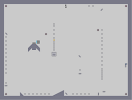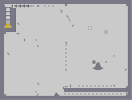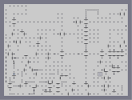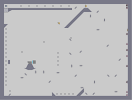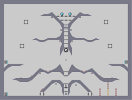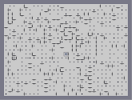Paratroopa Paratroopa 2: Behind Enemy Lines Secret Passageway... Paratroopa 3: The Great Escape Deep Forest Haunted Corridoors

Pages: (0)

### This is cool

This is great 4.5/5

### :D

i like this one :) its quite snazzy :P 4 easily :)